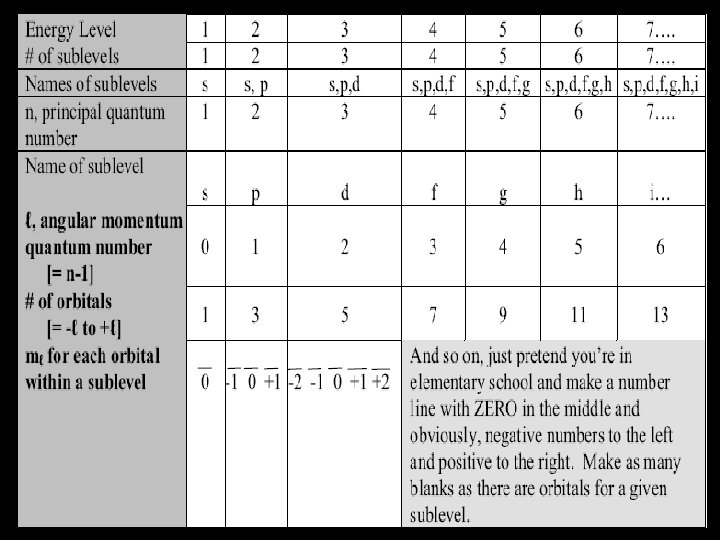Скачать презентацию Homework 10 1 Worksheet Quantum Numbers 2

9ab3fe67cb90cc206218b8f8547bc7aa.ppt

• Количество слайдов: 68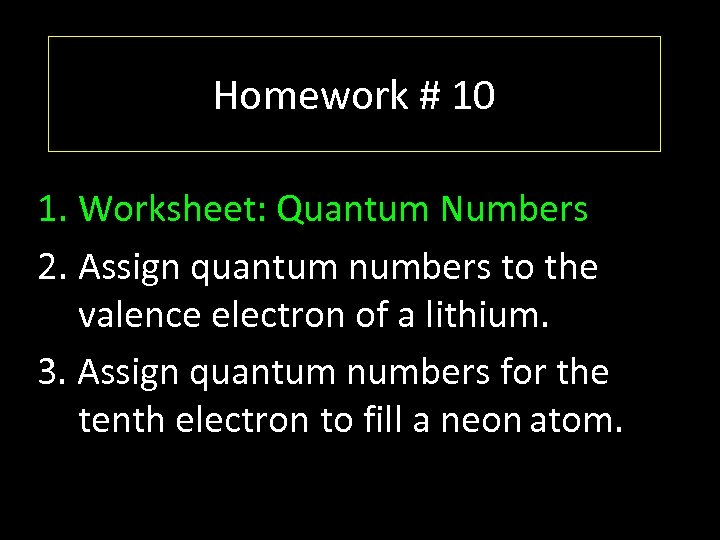Homework # 10 1. Worksheet: Quantum Numbers 2. Assign quantum numbers to the valence electron of a lithium. 3. Assign quantum numbers for the tenth electron to fill a neon atom.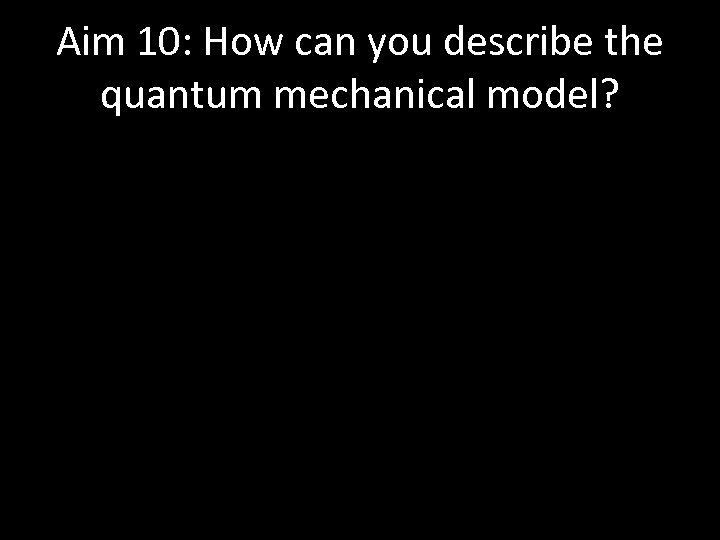Aim 10: How can you describe the quantum mechanical model?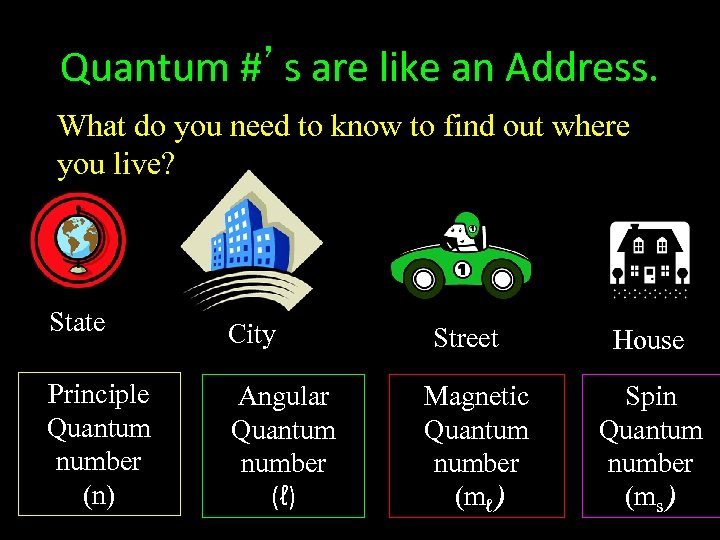Quantum #’s are like an Address. What do you need to know to find out where you live? State City Principle Quantum number (n) Angular Quantum number (ℓ) Street Magnetic Quantum number (mℓ) House Spin Quantum number (ms)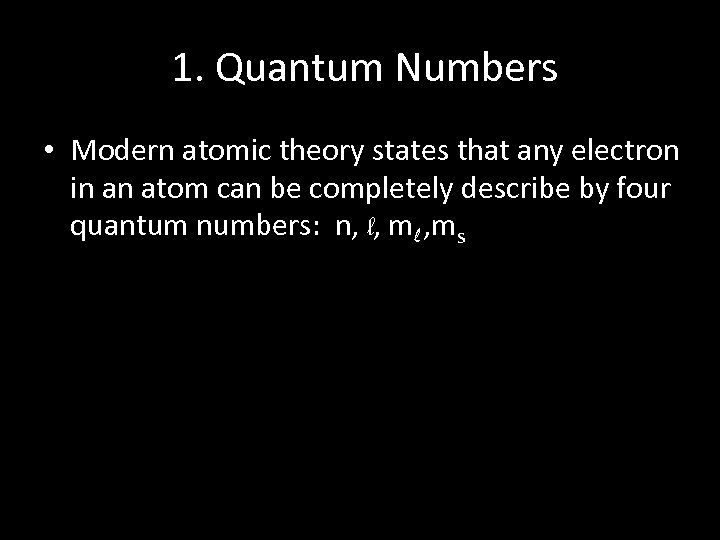1. Quantum Numbers • Modern atomic theory states that any electron in an atom can be completely describe by four quantum numbers: n, l, ml , ms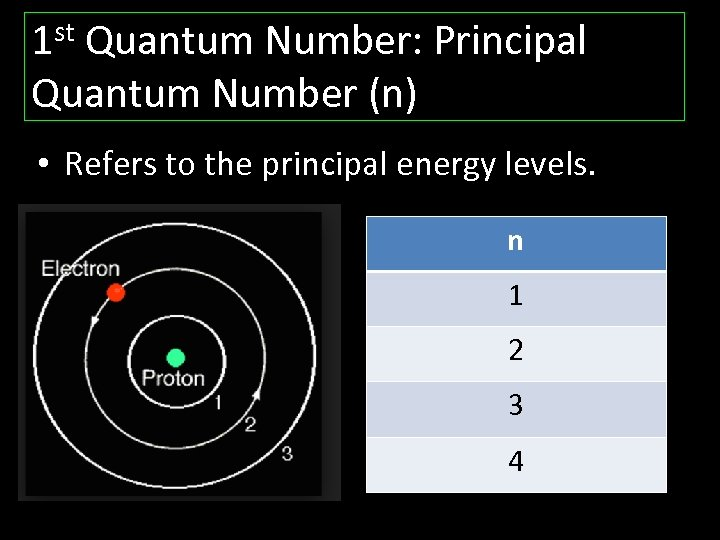1 st Quantum Number: Principal Quantum Number (n) • Refers to the principal energy levels. n 1 2 3 4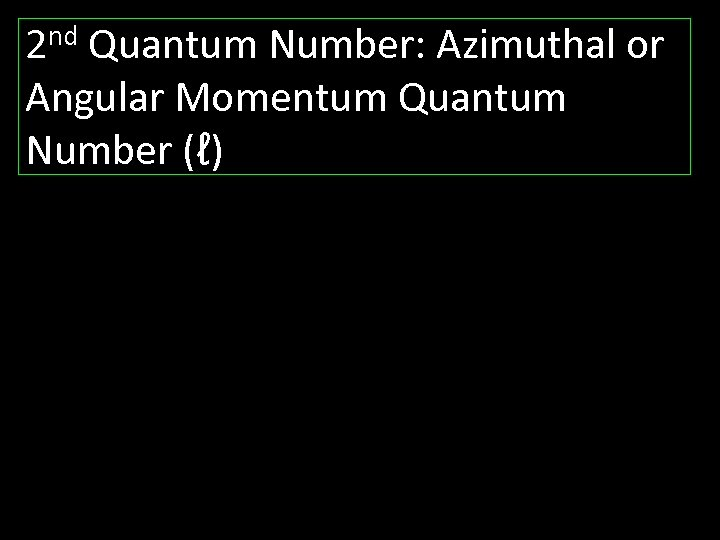2 nd Quantum Number: Azimuthal or Angular Momentum Quantum Number (ℓ)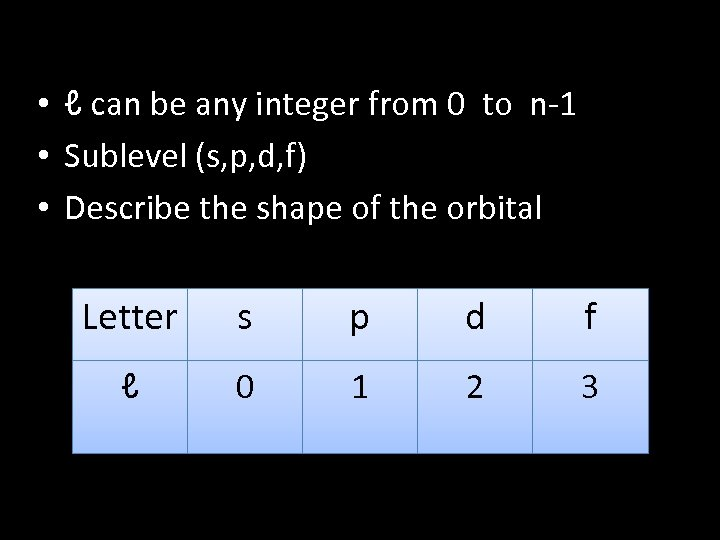• ℓ can be any integer from 0 to n-1 • Sublevel (s, p, d, f) • Describe the shape of the orbital Letter s p d f ℓ 0 1 2 3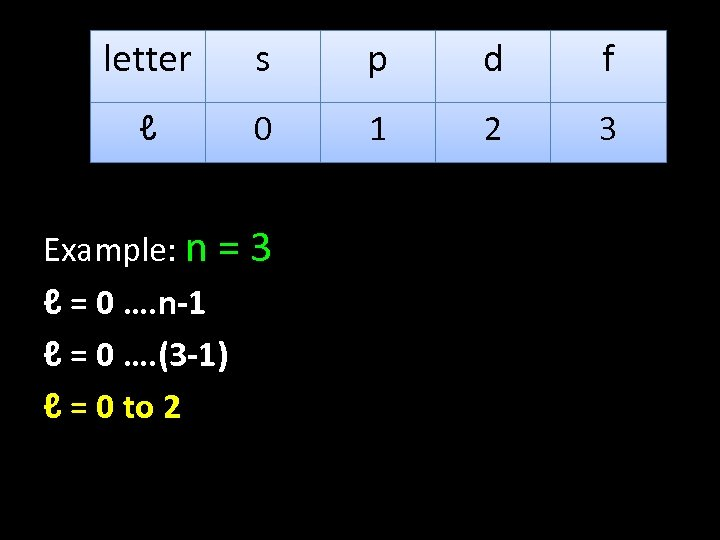letter s p d f ℓ 0 1 2 3 Example: n = 3 ℓ = 0 …. n-1 ℓ = 0 …. (3 -1) ℓ = 0 to 2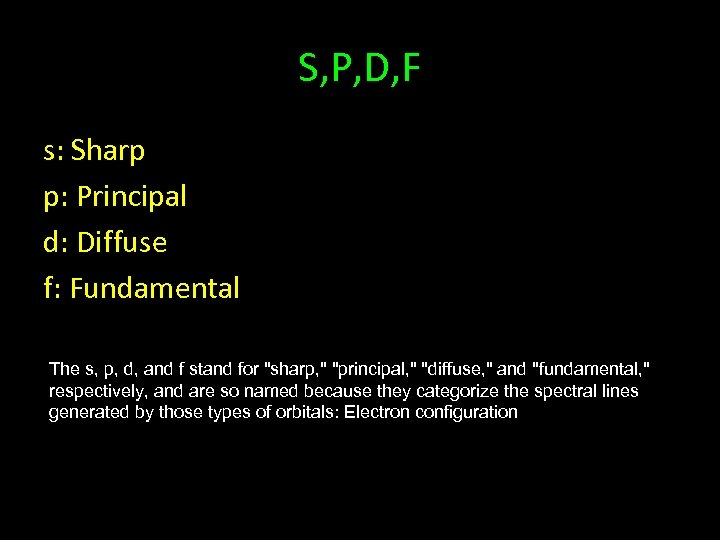S, P, D, F s: Sharp p: Principal d: Diffuse f: Fundamental The s, p, d, and f stand for "sharp, " "principal, " "diffuse, " and "fundamental, " respectively, and are so named because they categorize the spectral lines generated by those types of orbitals: Electron configuration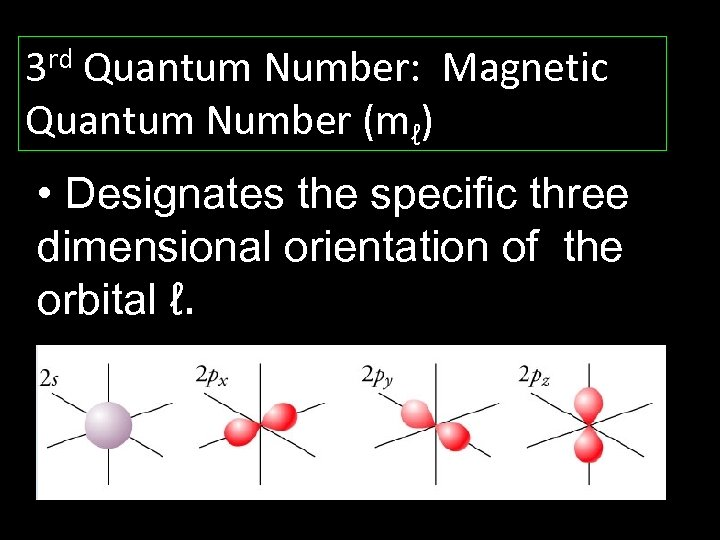3 rd Quantum Number: Magnetic Quantum Number (mℓ) • Designates the specific three dimensional orientation of the orbital ℓ.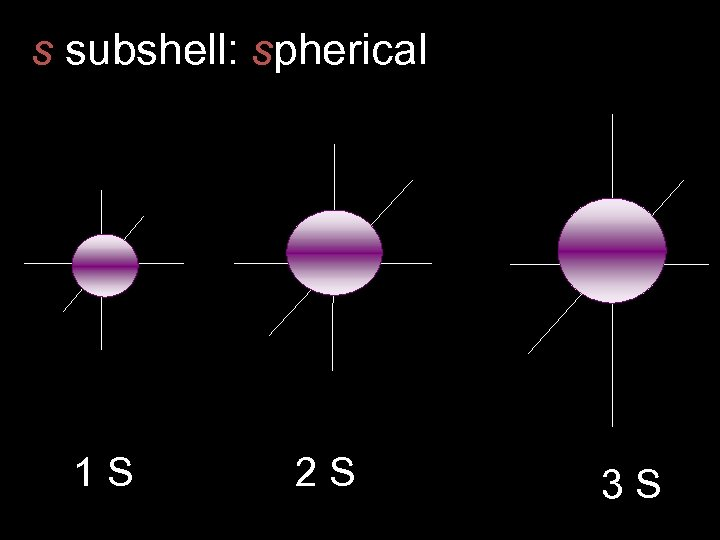s subshell: spherical 1 S 2 S 3 S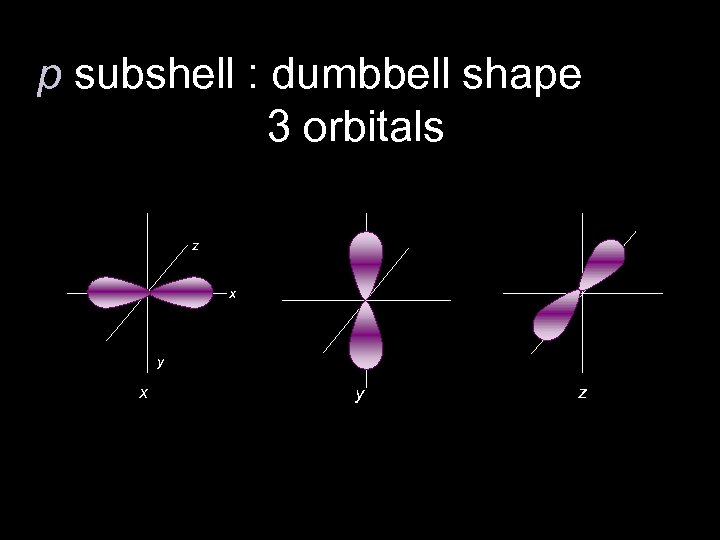p subshell : dumbbell shape 3 orbitals z x y z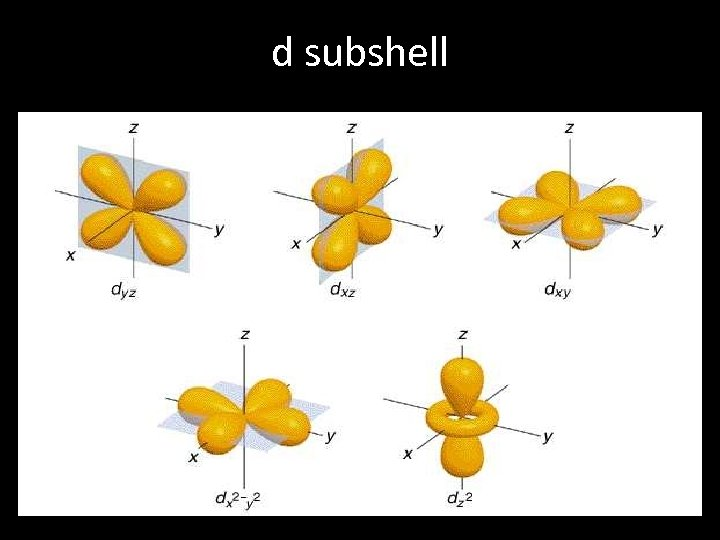d subshell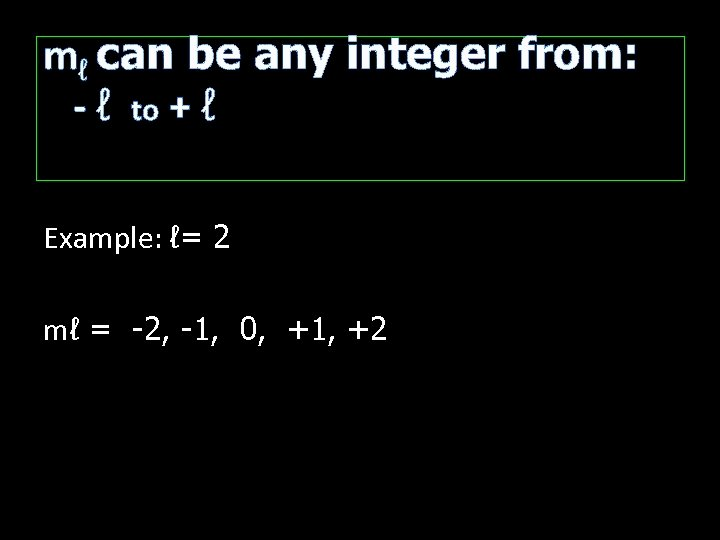mℓ can be any integer from: - ℓ to + ℓ Example: ℓ= 2 mℓ = -2, -1, 0, +1, +2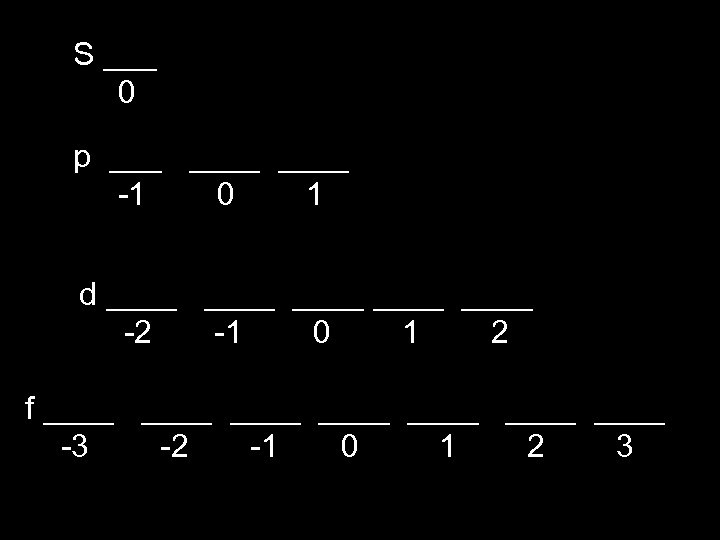S ___ 0 p ____ -1 0 1 d ____ ____ -2 -1 0 1 2 f ____ ____ -3 -2 -1 0 1 2 3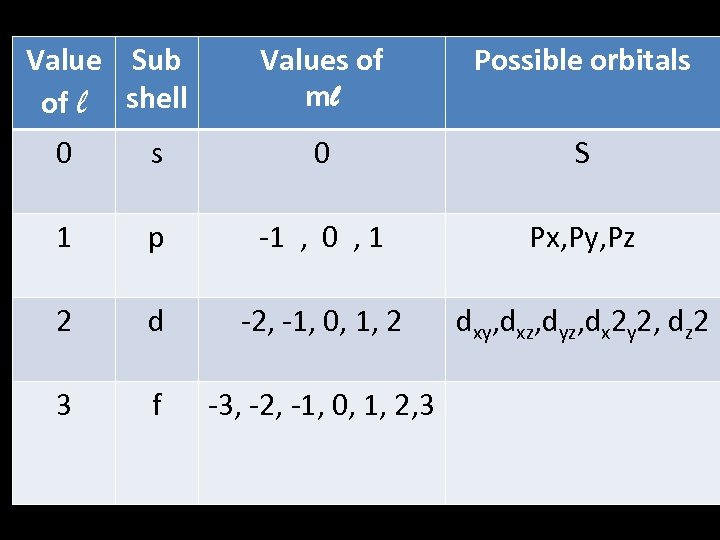Value Sub of l shell Values of ml Possible orbitals 0 S 1 p -1 , 0 , 1 Px, Py, Pz 2 d -2, -1, 0, 1, 2 dxy, dxz, dyz, dx 2 y 2, dz 2 3 f -3, -2, -1, 0, 1, 2, 3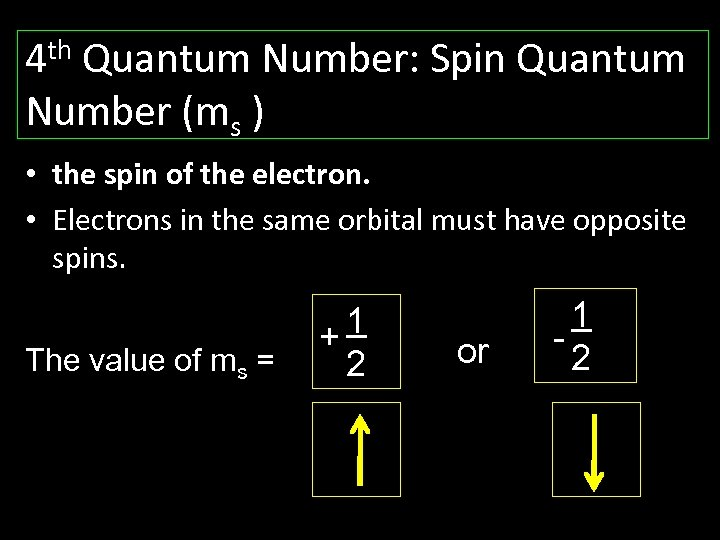th 4 Quantum Number: Spin Quantum Number (ms ) • the spin of the electron. • Electrons in the same orbital must have opposite spins. The value of ms = 1 + 2 or 1 2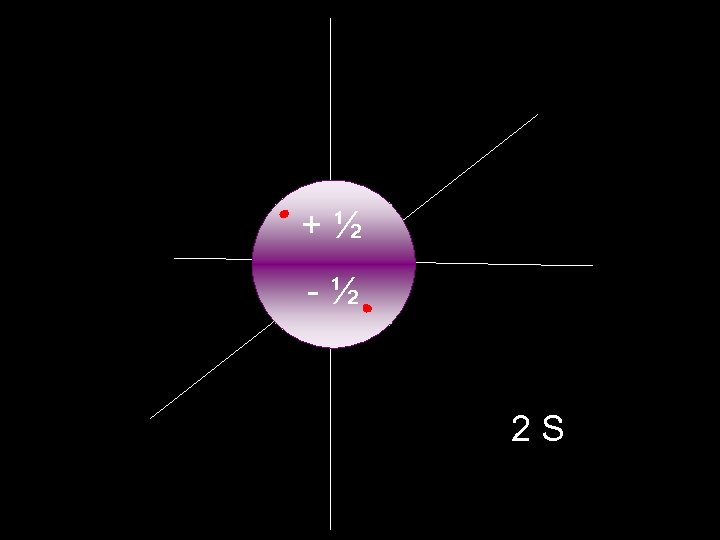+½ -½ 2 S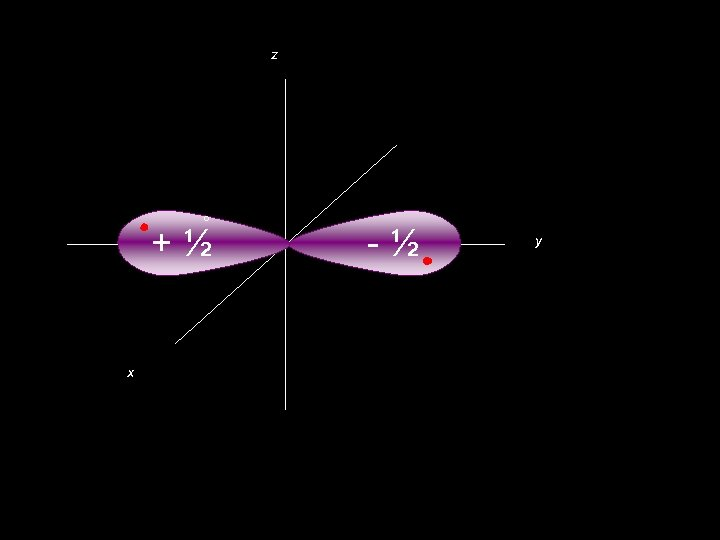z ° +½ x -½ y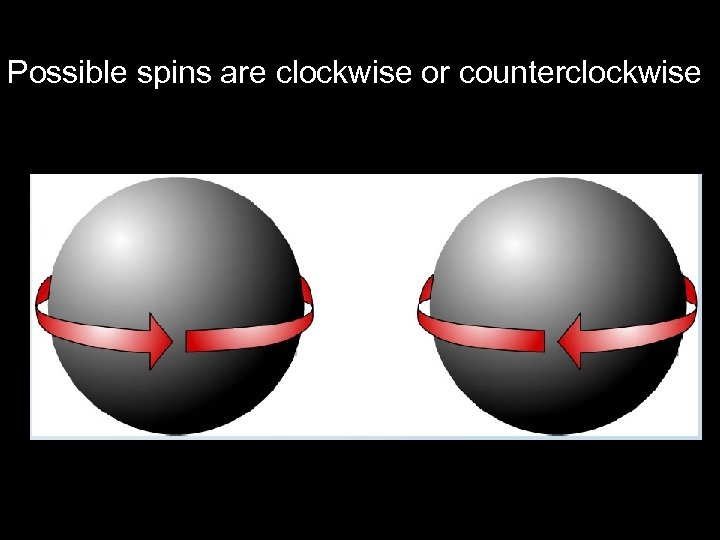Possible spins are clockwise or counterclockwise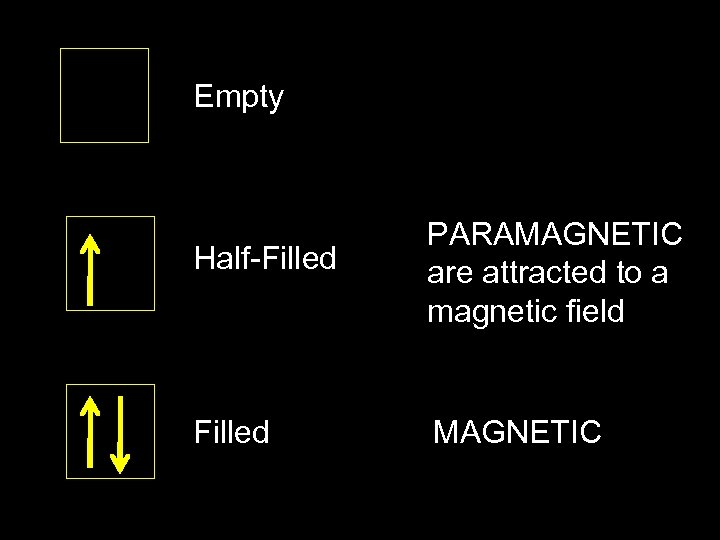Empty Half-Filled PARAMAGNETIC are attracted to a magnetic field Filled MAGNETIC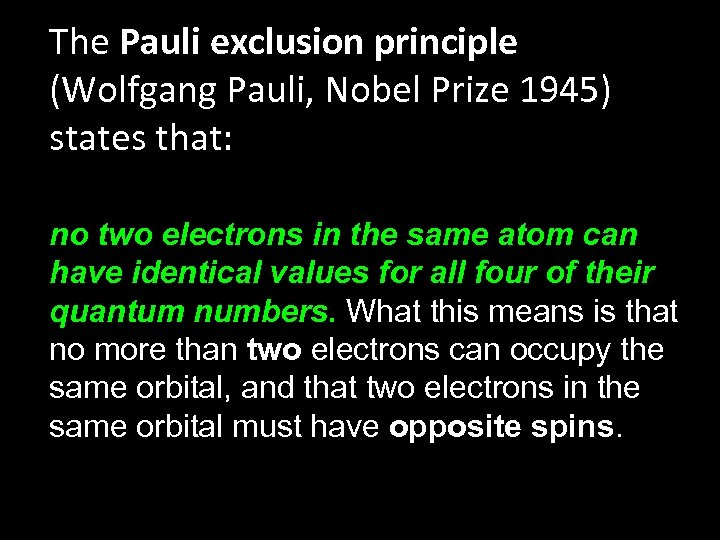The Pauli exclusion principle (Wolfgang Pauli, Nobel Prize 1945) states that: no two electrons in the same atom can have identical values for all four of their quantum numbers. What this means is that no more than two electrons can occupy the same orbital, and that two electrons in the same orbital must have opposite spins.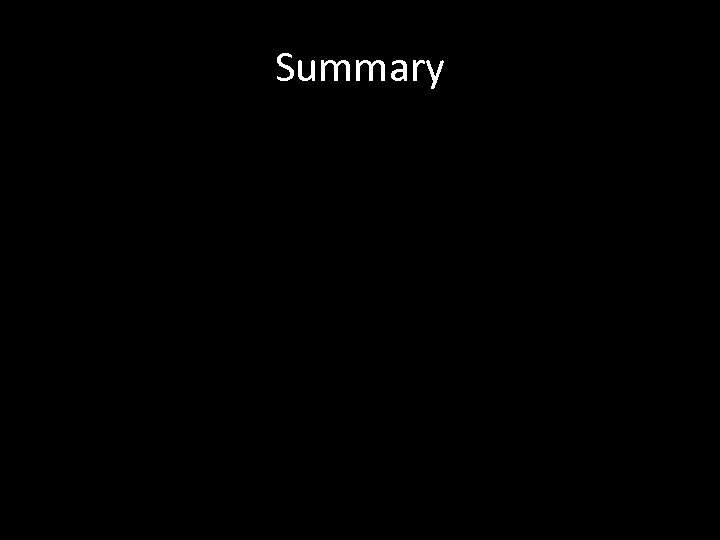SummaryQuantum Numbers n, PEL l, ms ORBITAL SPIN SUBLEVEL SHAPE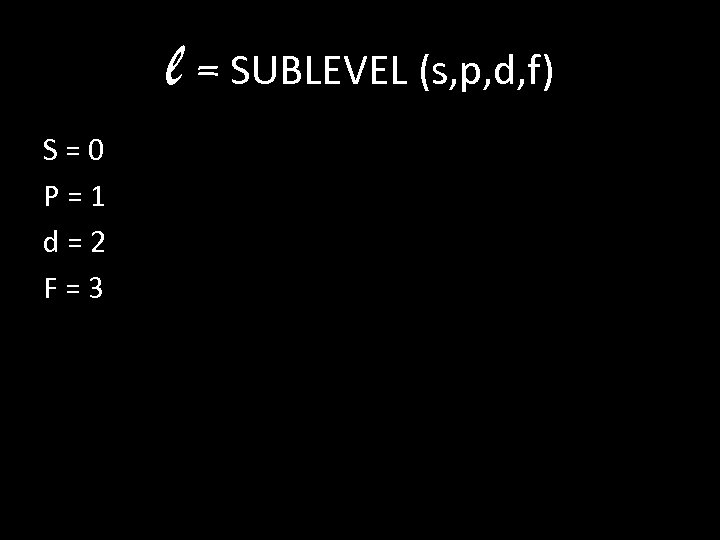l = SUBLEVEL (s, p, d, f) S=0 P=1 d=2 F=3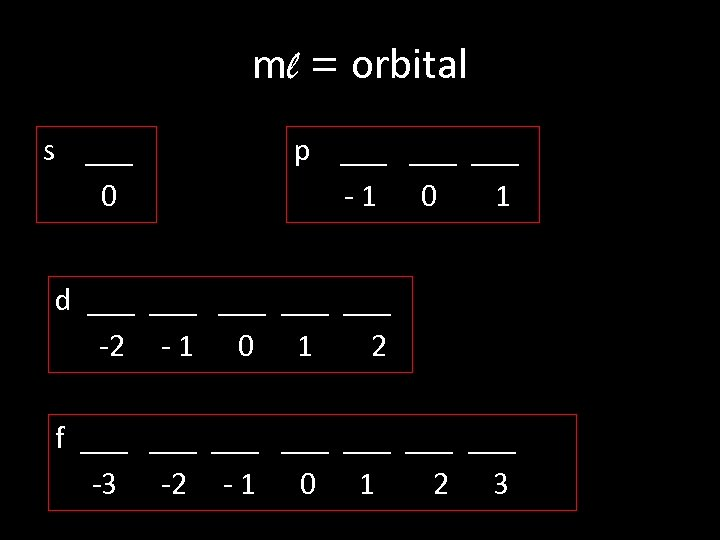ml = orbital s ___ 0 p ___ ___ -1 0 1 d ___ ___ ___ -2 - 1 0 1 2 f ___ ___ -3 -2 - 1 0 1 2 3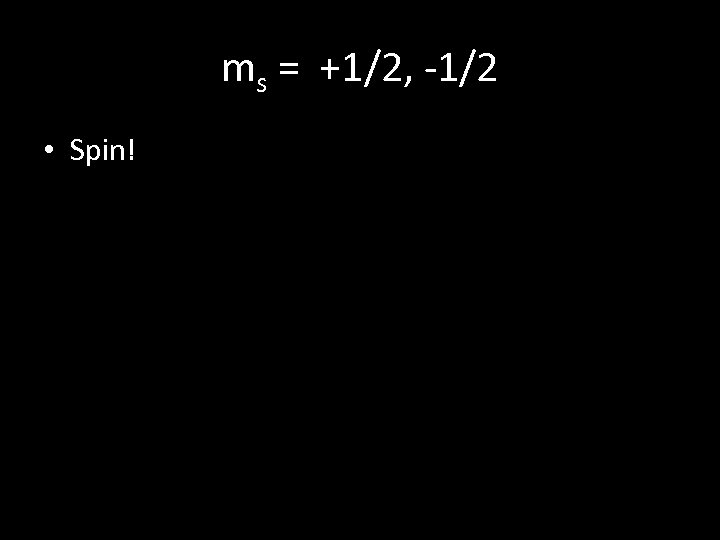ms = +1/2, -1/2 • Spin!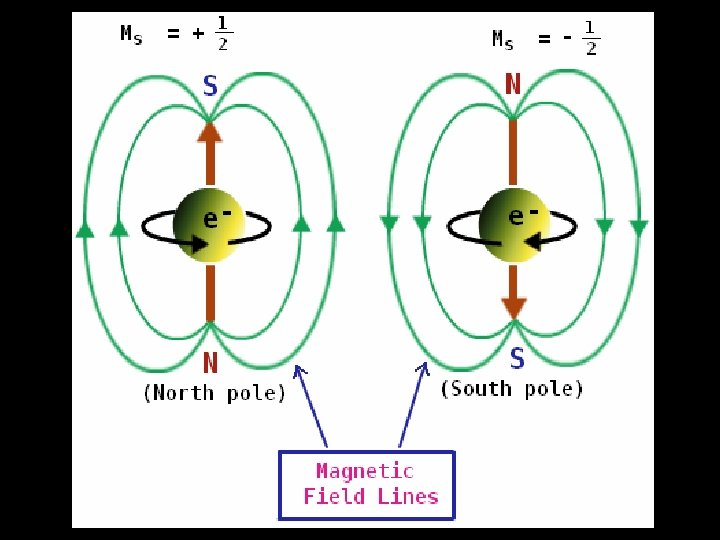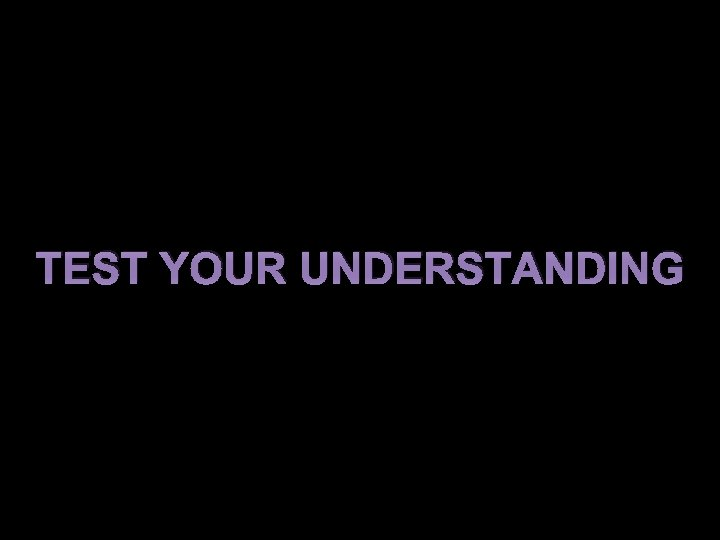TEST YOUR UNDERSTANDING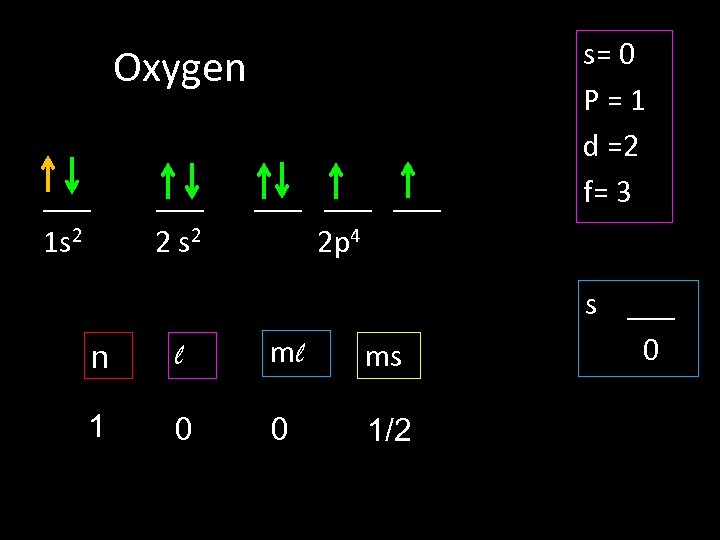Oxygen ___ 1 s 2 ___ 2 s 2 ___ ___ 2 p 4 n l ml ms 1 0 0 1/2 s= 0 P=1 d =2 f= 3 s ___ 0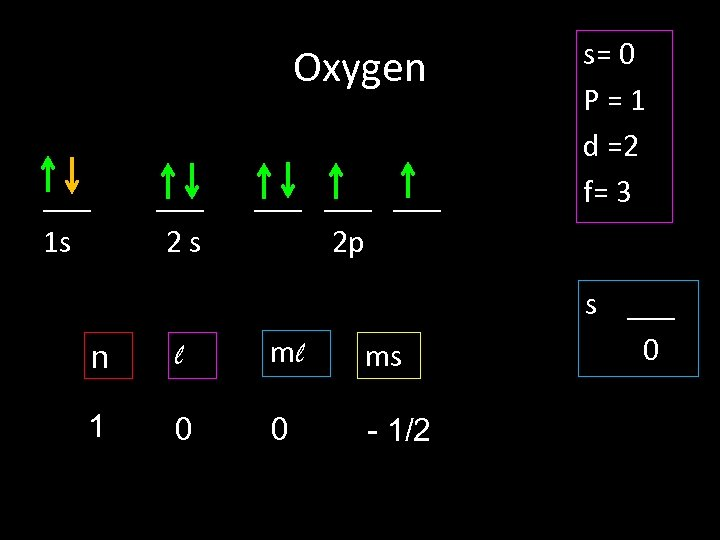Oxygen ___ 1 s ___ 2 s ___ ___ 2 p n l ml ms 1 0 0 - 1/2 s= 0 P=1 d =2 f= 3 s ___ 0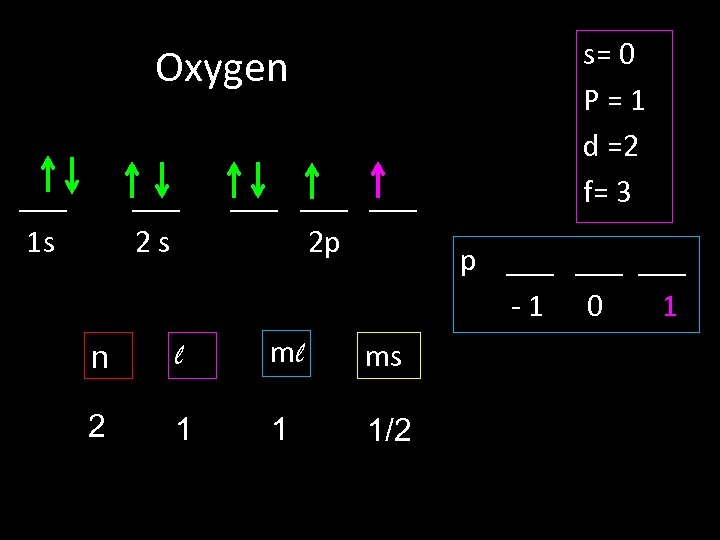Oxygen ___ 1 s ___ 2 s ___ ___ 2 p n l ml ms 2 1 1 1/2 s= 0 P=1 d =2 f= 3 p ___ ___ -1 0 1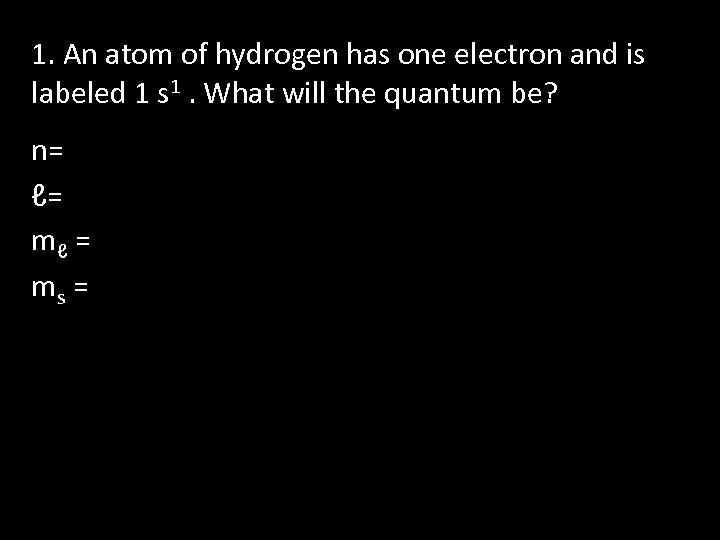1. An atom of hydrogen has one electron and is labeled 1 s 1. What will the quantum be? n= ℓ= mℓ = ms =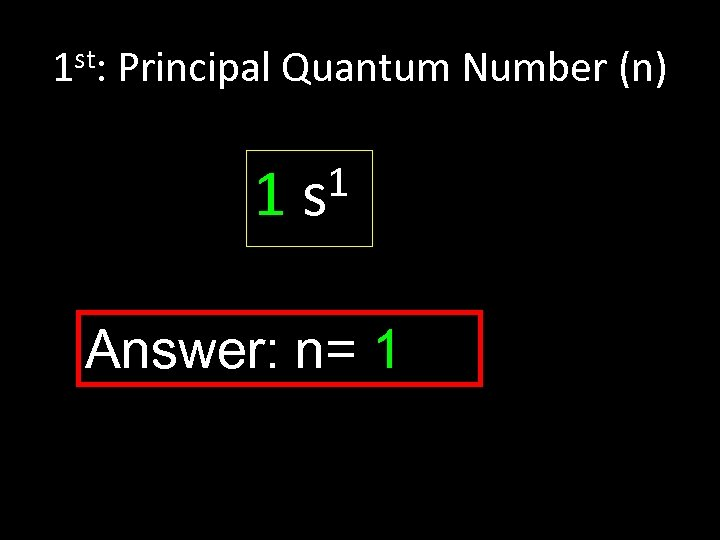1 st: Principal Quantum Number (n) 1 1 s Answer: n= 1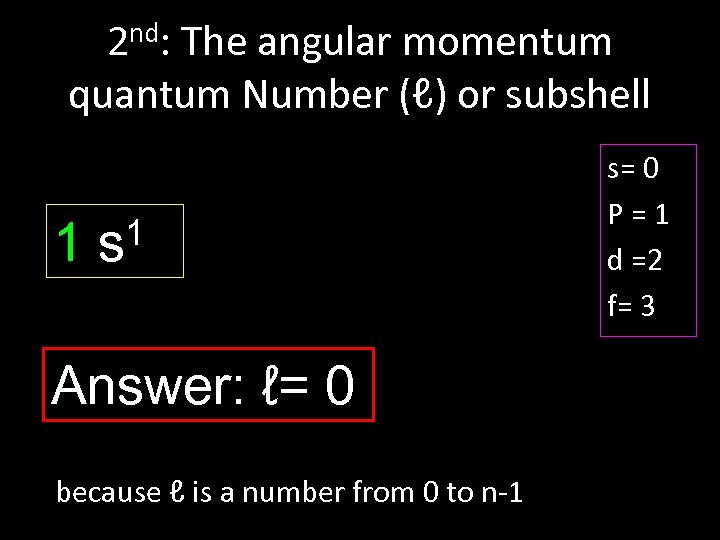2 nd: The angular momentum quantum Number (ℓ) or subshell 1 1 s Answer: ℓ= 0 because ℓ is a number from 0 to n-1 s= 0 P=1 d =2 f= 3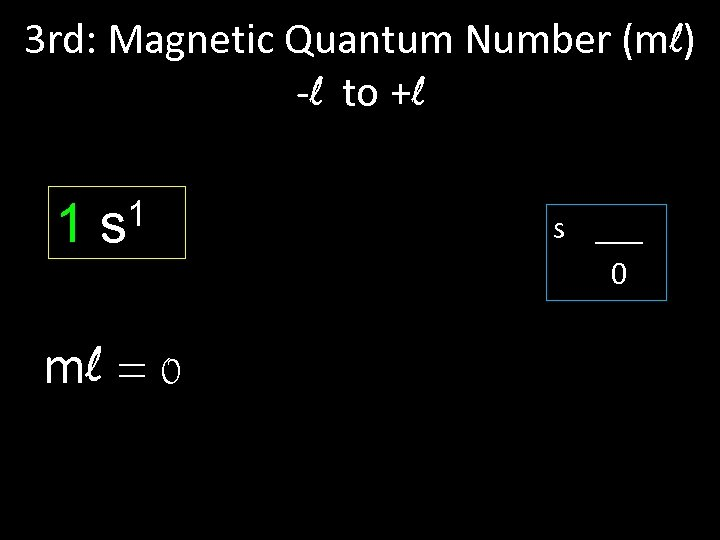3 rd: Magnetic Quantum Number (ml) -l to +l 1 1 s ml = 0 s ___ 0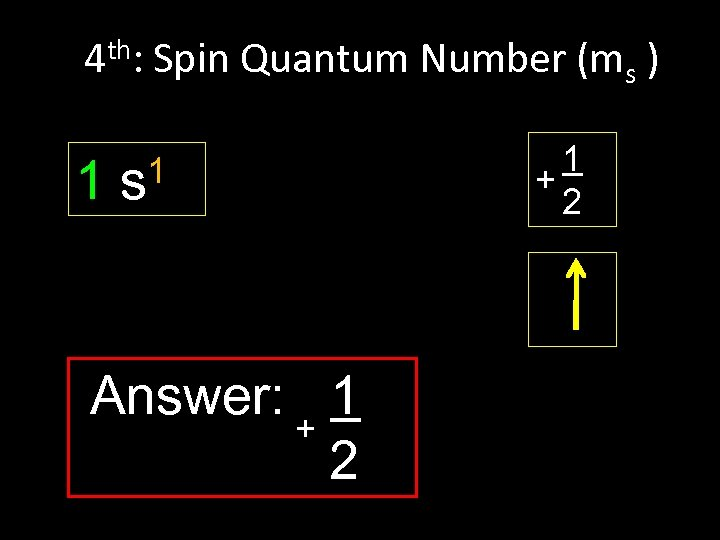4 th: Spin Quantum Number (ms ) 1 1 s Answer: + 1 2 1 + 2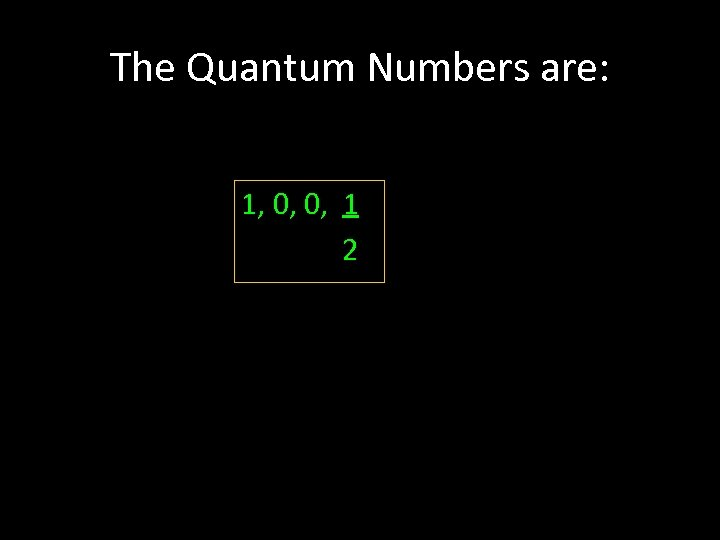The Quantum Numbers are: 1, 0, 0, 1 2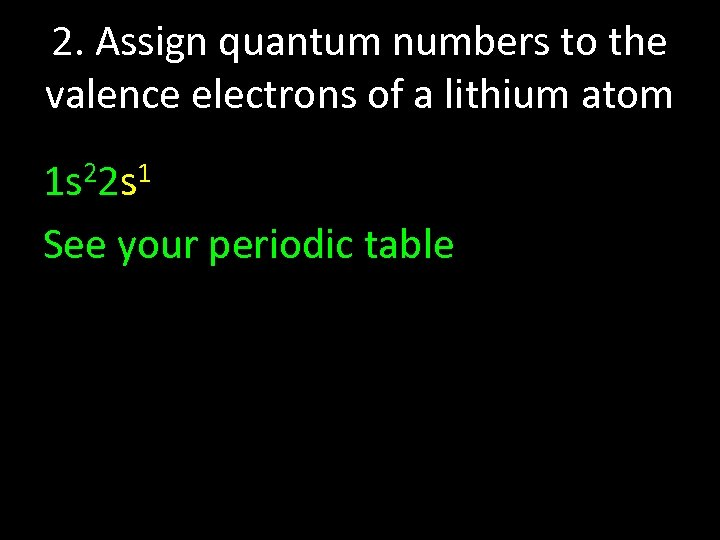2. Assign quantum numbers to the valence electrons of a lithium atom 1 s 22 s 1 See your periodic table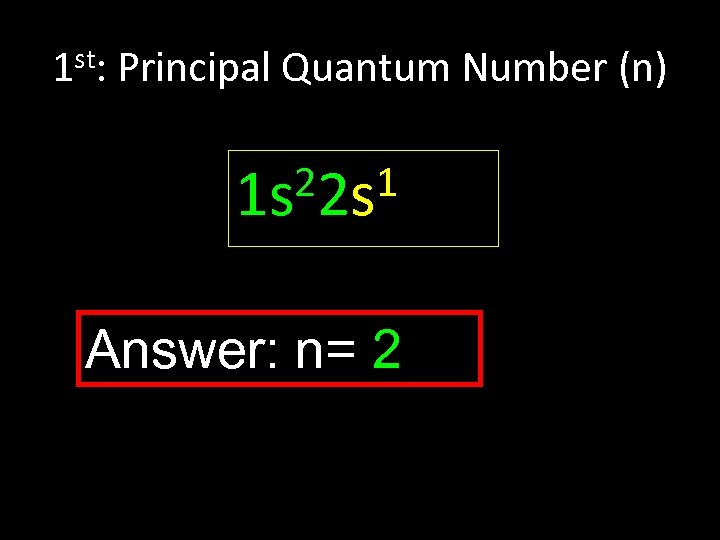1 st: Principal Quantum Number (n) 22 s 1 1 s Answer: n= 2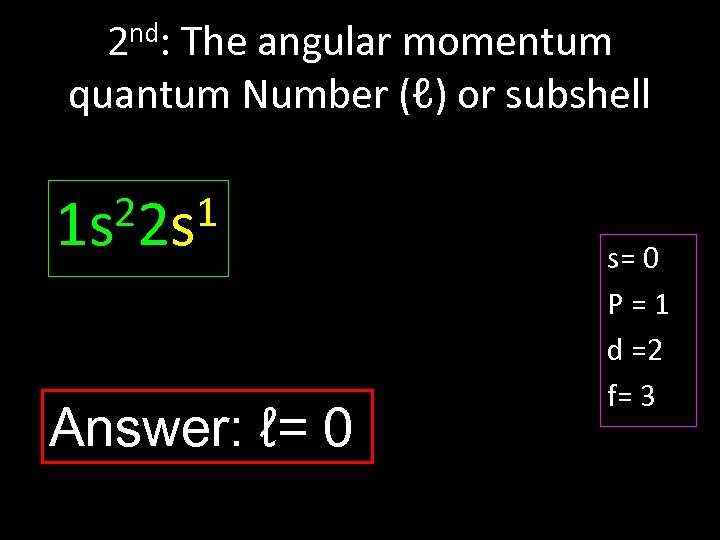2 nd: The angular momentum quantum Number (ℓ) or subshell 22 s 1 1 s Answer: ℓ= 0 s= 0 P=1 d =2 f= 3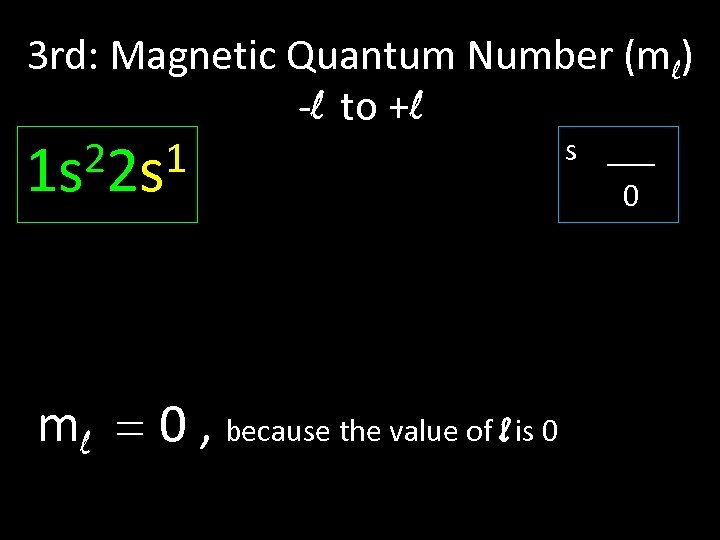3 rd: Magnetic Quantum Number (ml) -l to +l s ___ 22 s 1 1 s 0 ml = 0 , because the value of l is 0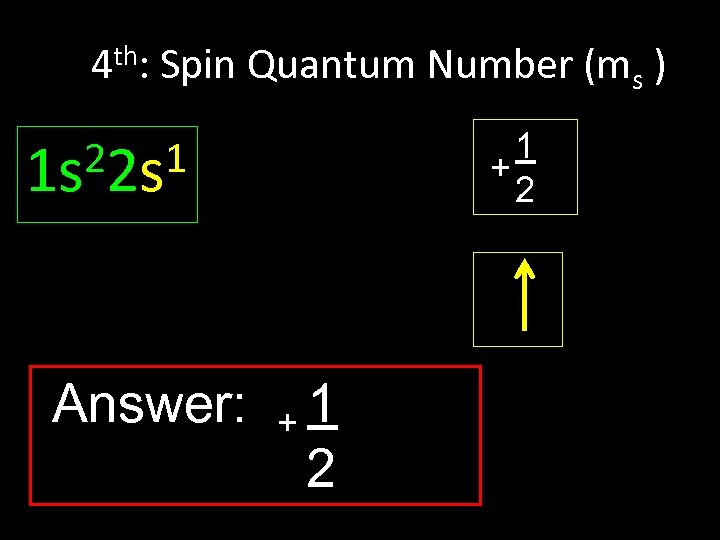4 th: Spin Quantum Number (ms ) 1 + 2 22 s 1 1 s Answer: +1 2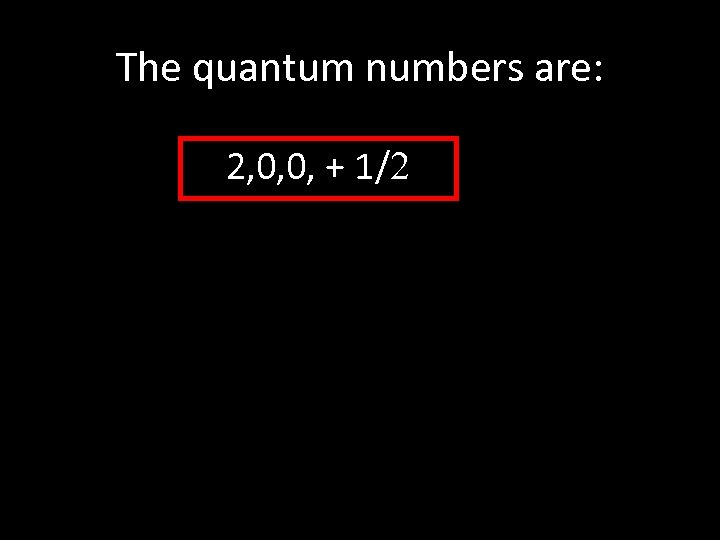The quantum numbers are: 2, 0, 0, + 1/2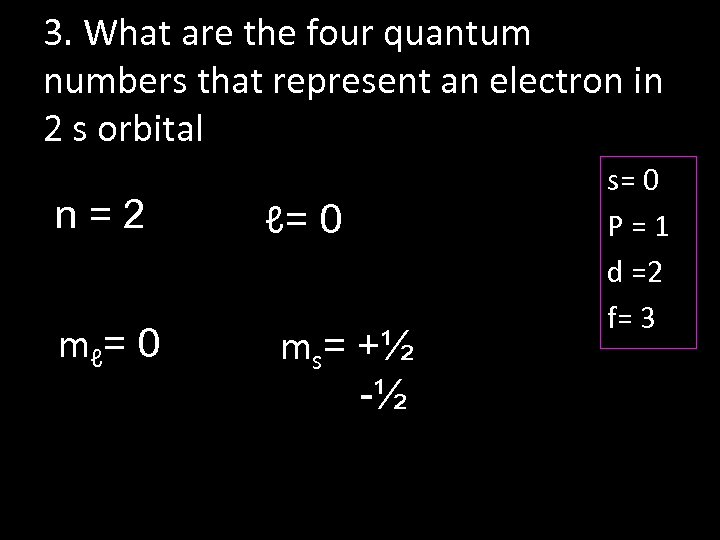3. What are the four quantum numbers that represent an electron in 2 s orbital n=2 m ℓ= 0 ms= +½ -½ s= 0 P=1 d =2 f= 3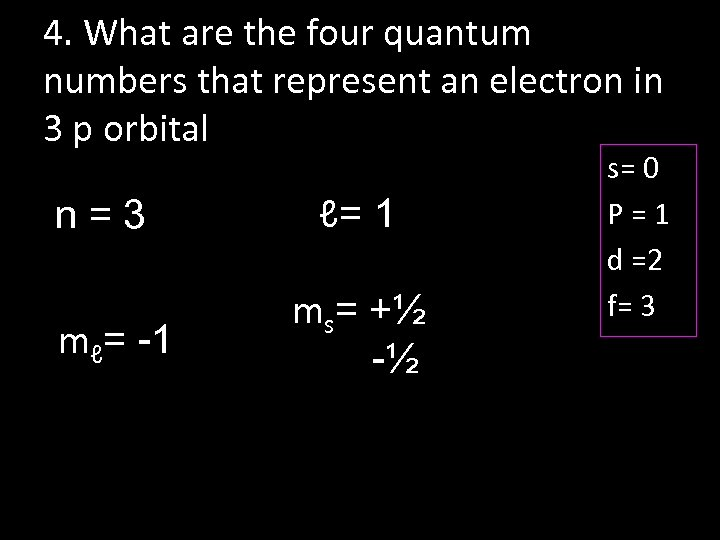4. What are the four quantum numbers that represent an electron in 3 p orbital n=3 mℓ= -1 ℓ= 1 ms= +½ -½ s= 0 P=1 d =2 f= 3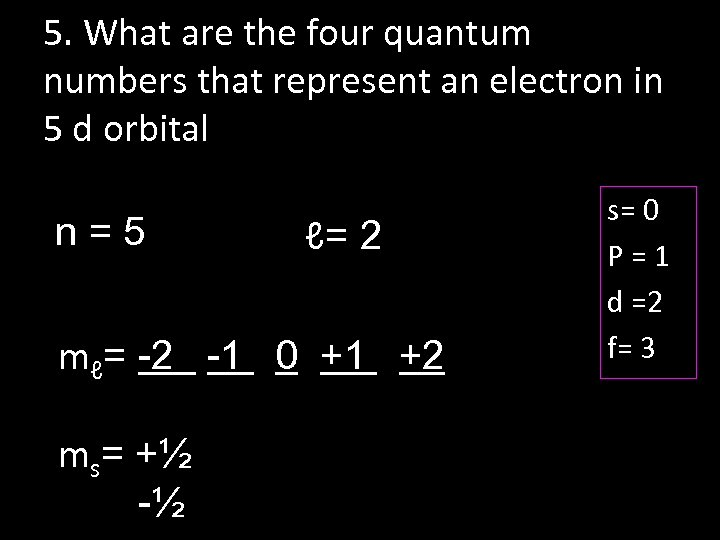5. What are the four quantum numbers that represent an electron in 5 d orbital n=5 ℓ= 2 mℓ= -2 -1 0 +1 +2 ms= +½ -½ s= 0 P=1 d =2 f= 3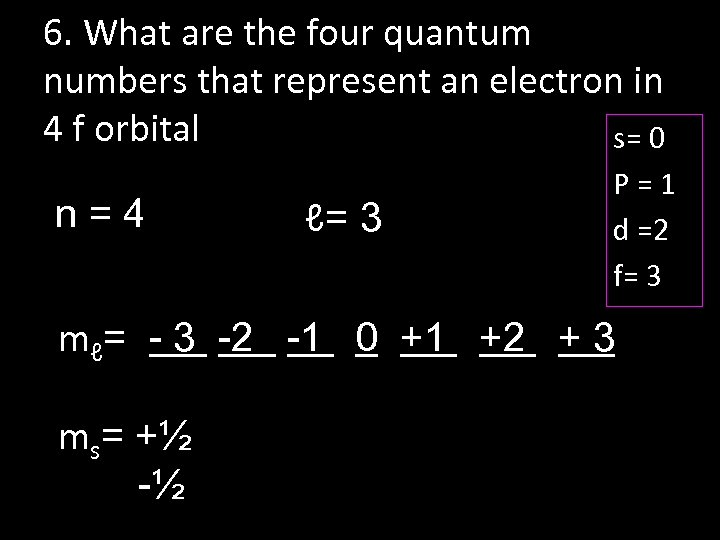6. What are the four quantum numbers that represent an electron in 4 f orbital s= 0 n=4 ℓ= 3 P=1 d =2 f= 3 mℓ= - 3 -2 -1 0 +1 +2 + 3 ms= +½ -½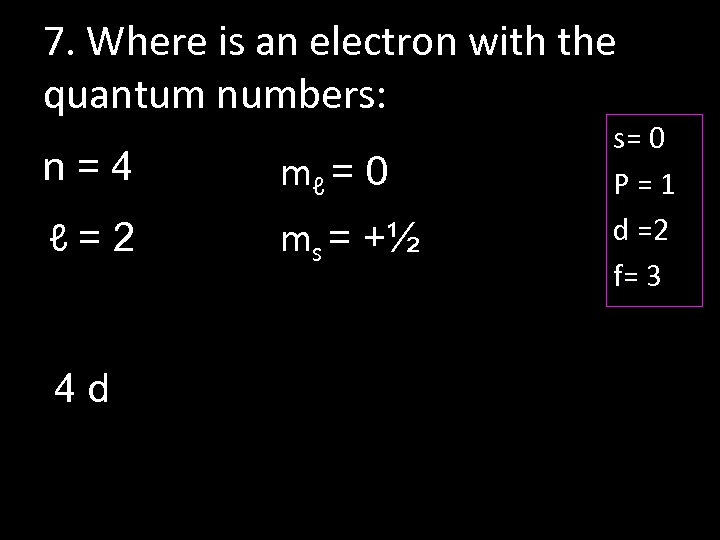7. Where is an electron with the quantum numbers: n=4 mℓ = 0 ℓ=2 ms = +½ 4 d s= 0 P=1 d =2 f= 3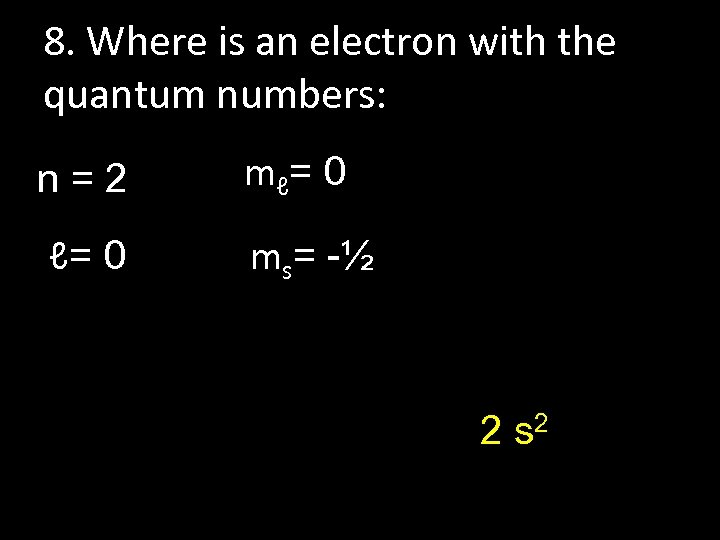8. Where is an electron with the quantum numbers: n=2 m ℓ= 0 ms= -½ 2 s 2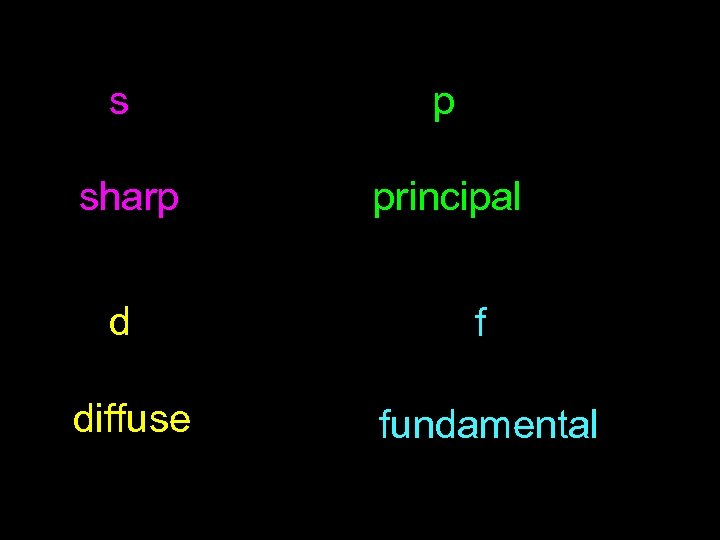s p sharp principal d f diffuse fundamental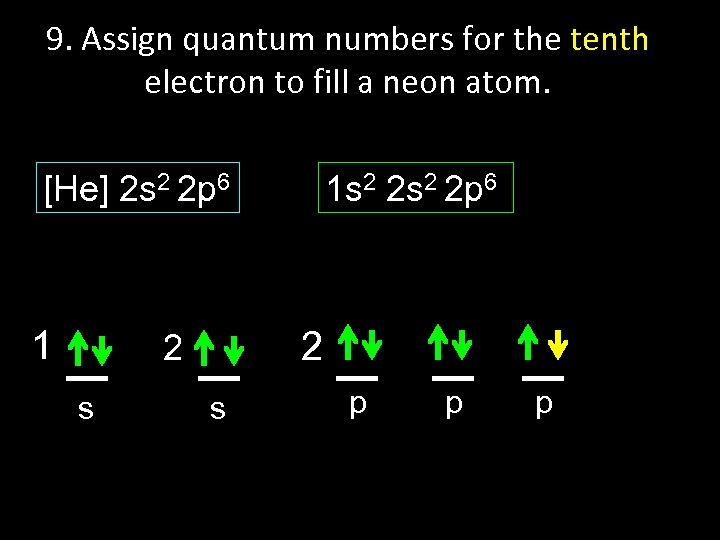9. Assign quantum numbers for the tenth electron to fill a neon atom. [He] 2 s 2 2 p 6 1 2 2 s 1 s 2 2 p 6 s p p p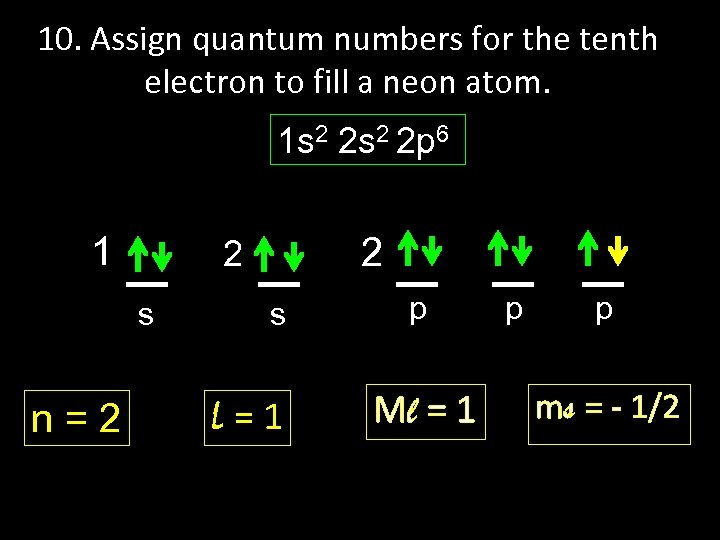10. Assign quantum numbers for the tenth electron to fill a neon atom. 1 s 2 2 p 6 1 s n=2 2 2 s L=1 p Ml = 1 p p ms = - 1/2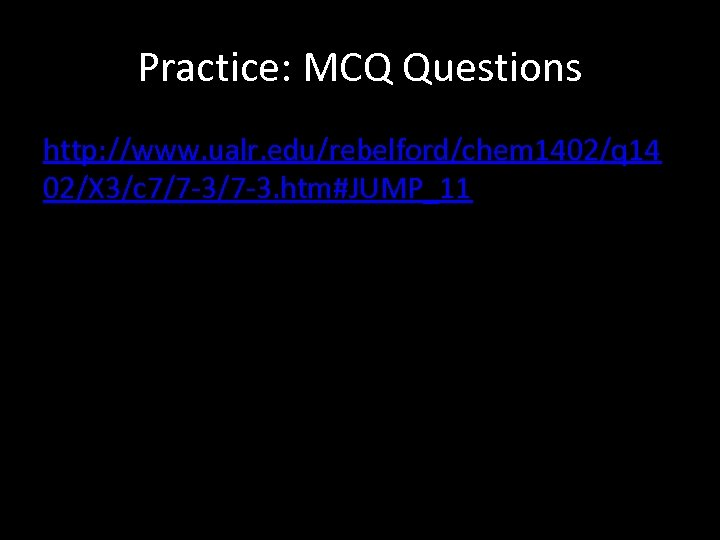Practice: MCQ Questions http: //www. ualr. edu/rebelford/chem 1402/q 14 02/X 3/c 7/7 -3. htm#JUMP_11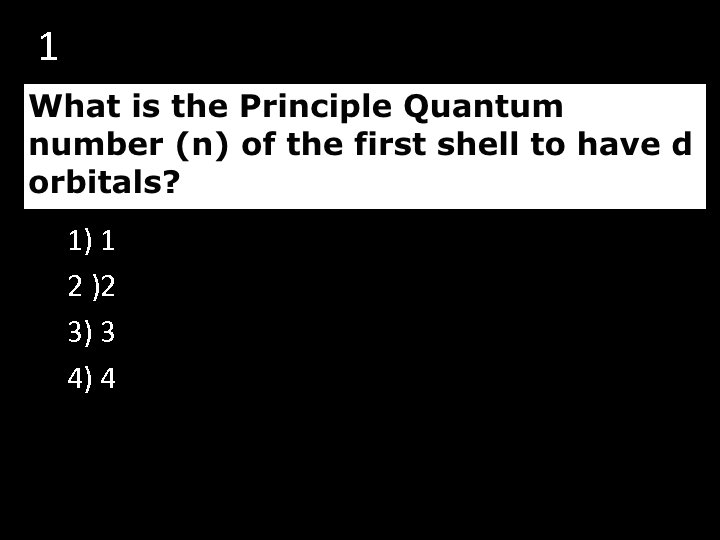1 1) 1 2 )2 3) 3 4) 4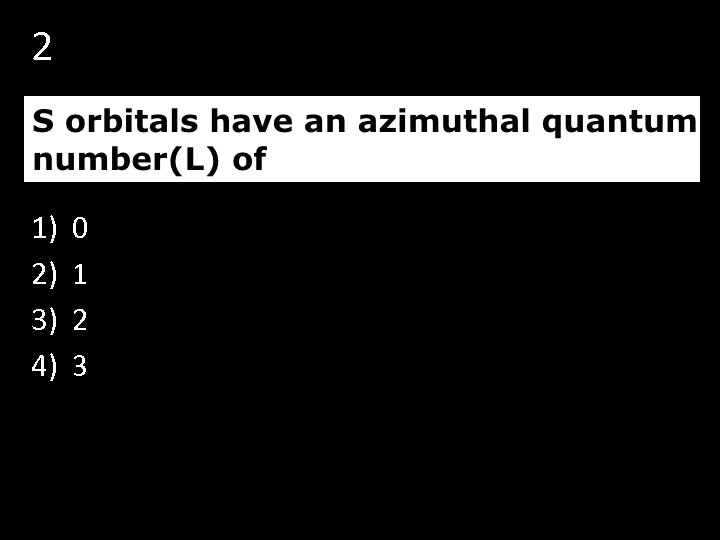2 1) 2) 3) 4) 0 1 2 3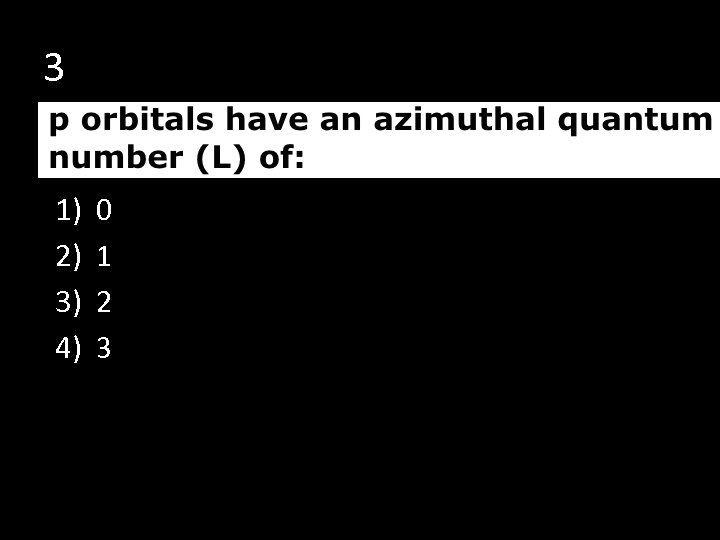3 1) 2) 3) 4) 0 1 2 3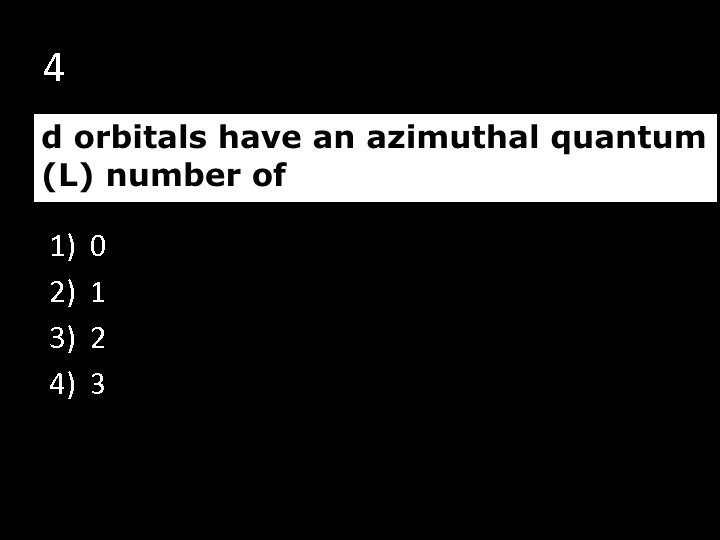4 1) 2) 3) 4) 0 1 2 3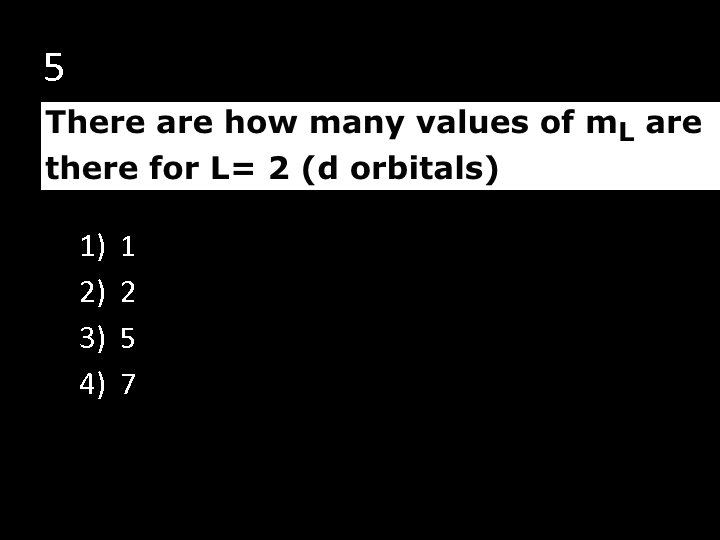5 1) 2) 3) 4) 1 2 5 7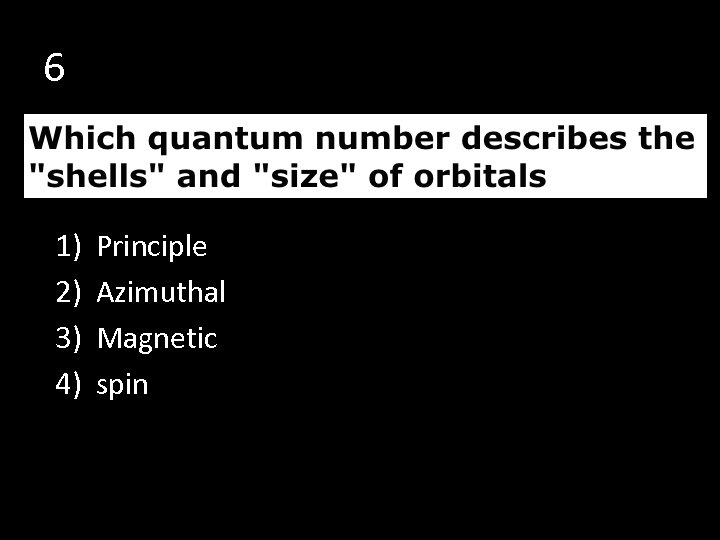6 1) 2) 3) 4) Principle Azimuthal Magnetic spin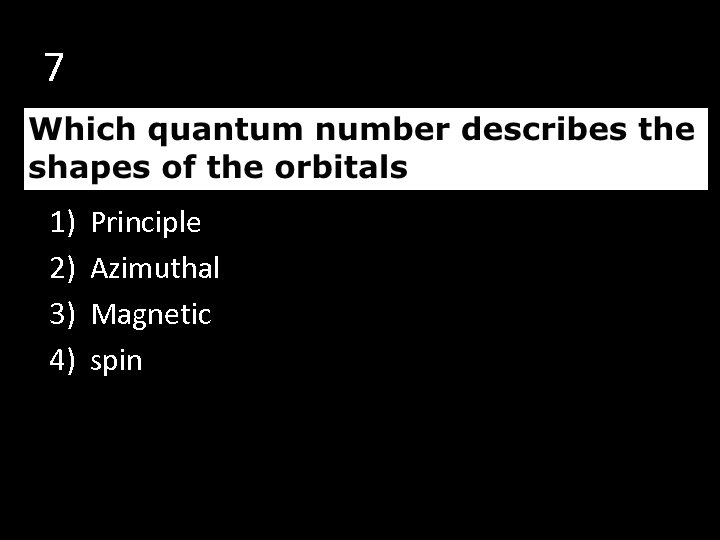7 1) 2) 3) 4) Principle Azimuthal Magnetic spin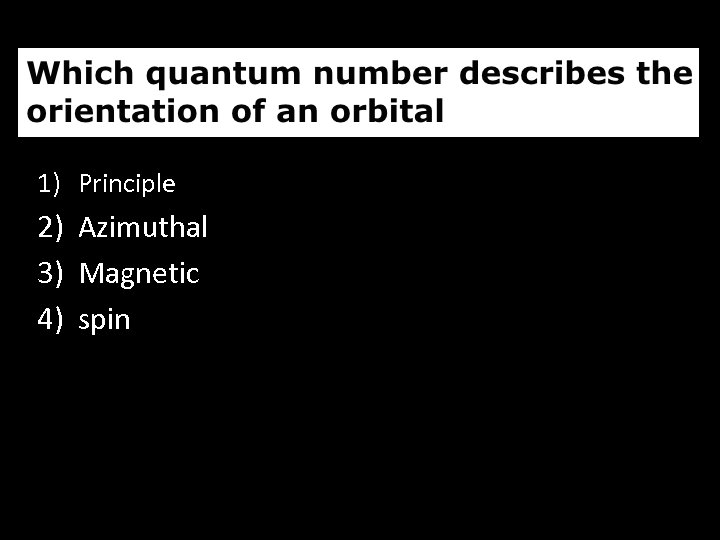1) Principle 2) Azimuthal 3) Magnetic 4) spin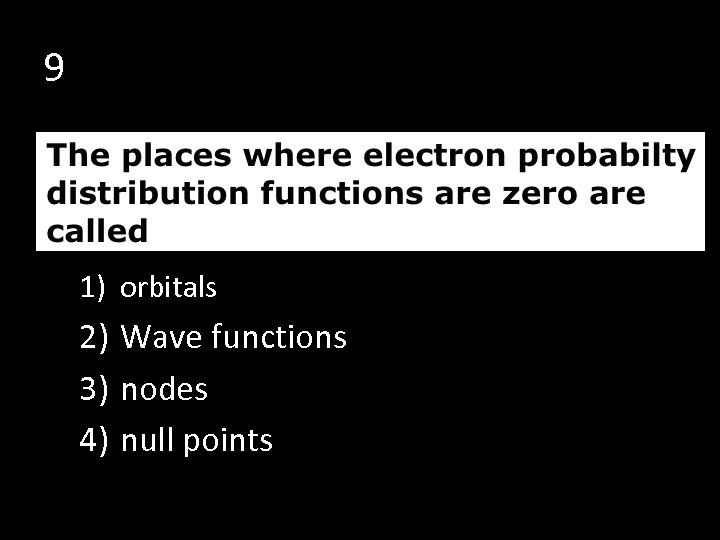9 1) orbitals 2) Wave functions 3) nodes 4) null points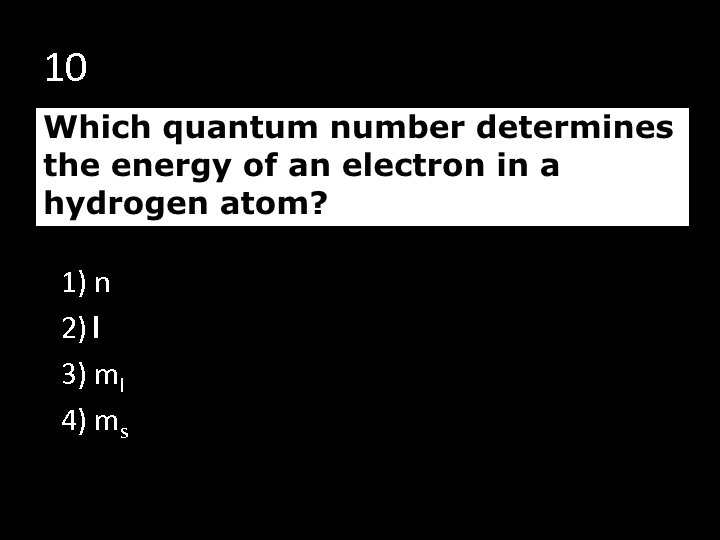10 1) n 2) l 3) ml 4) ms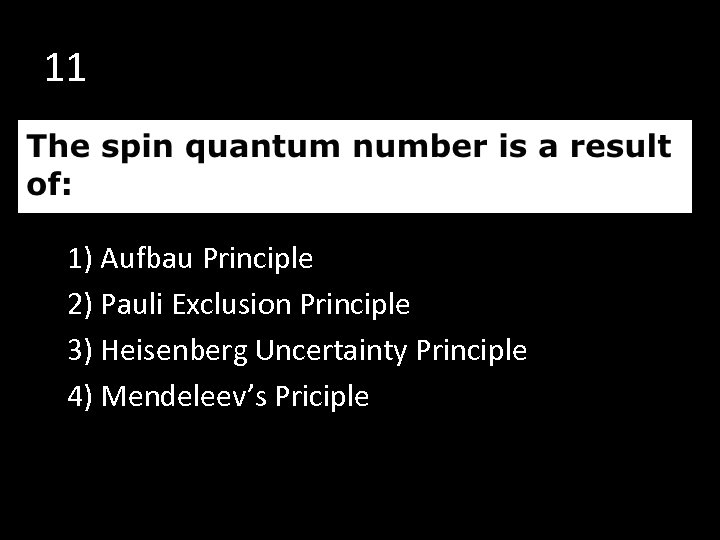11 1) Aufbau Principle 2) Pauli Exclusion Principle 3) Heisenberg Uncertainty Principle 4) Mendeleev’s PricipleEND OF THE SHOW !!!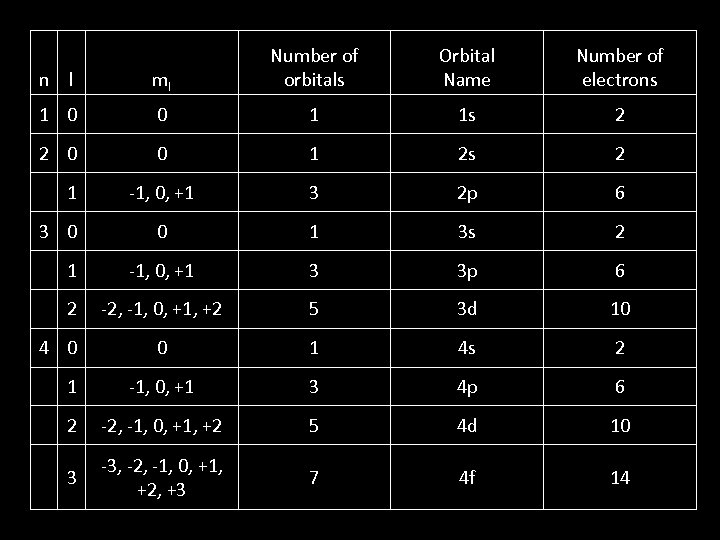l ml Number of orbitals 1 0 0 1 1 s 2 2 0 0 1 2 s 2 -1, 0, +1 3 2 p 6 0 1 3 s 2 1 -1, 0, +1 3 3 p 6 2 -2, -1, 0, +1, +2 5 3 d 10 0 1 4 s 2 1 -1, 0, +1 3 4 p 6 2 -2, -1, 0, +1, +2 5 4 d 10 3 -3, -2, -1, 0, +1, +2, +3 7 4 f 14 n 1 3 0 4 0 Orbital Name Number of electrons